# Quiz 4: Estimating Demand

The data below shows the per capita can consumption of soft drink per year related to price of six packs, per capita income, and mean temperature across the 48 adjoining states in the country US.Calculate average cans per capita across 48 contiguous states in the country US by entering the formula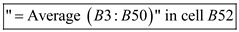. Calculate average 6 - pack price across 48 contiguous states in the country US by entering the formula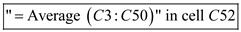. Calculate average income per capita across 48 contiguous states in the country US by entering the formula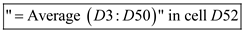. Calculate average mean temperature across 48 contiguous states in the country US by entering the formula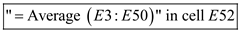. To run regression, select the "Data" tab and click "Data Analysis" in the "Analysis" grouping. Input the data of dependent variable in the "Input Y-Range" field, and then enter data of independent variables in the "Input X -Range" field for multiple columns. Click "OK" to create the analysis as below.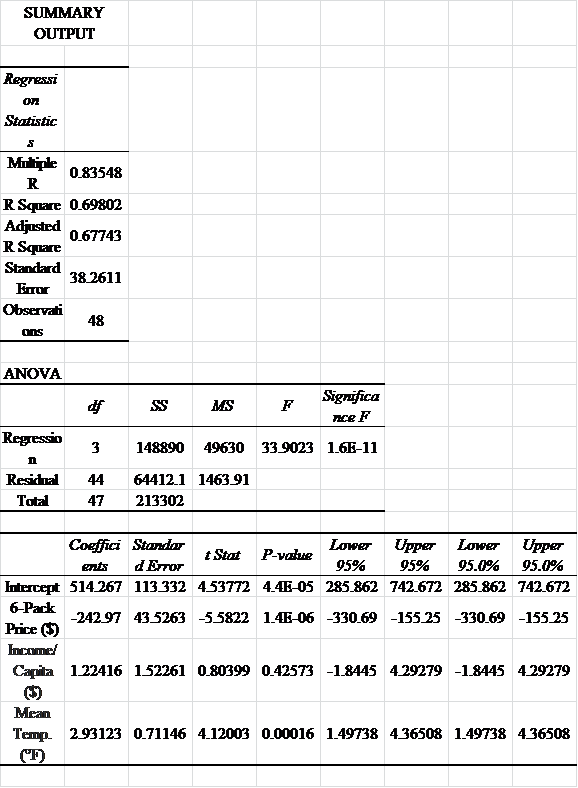The intercept is calculated as 514.267. The coefficients of 6-pack price, income per capita and mean temperature are - 242.97 , 1.22 and 2.93. Thus, the demand equation estimated using OLS is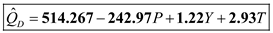.
a) The regression equation of the Sherwin-Williams Company's sales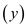on its selling price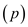is given as: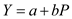Where,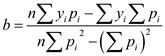and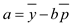The company's average sales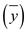are calculated as:The average selling price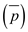is calculated as:Following calculations are done on the basis of the information given above.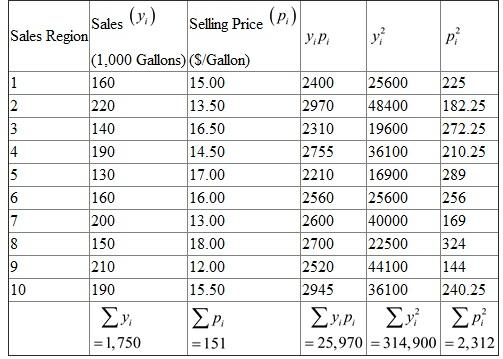For the above calculated values,And,Thus, the regression equation of the company's sales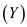on its selling price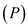is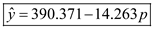. b) The value of intercept indicates that when the selling price is zero, the sales by Sherwin-Williams Company are as high as 390,371 gallons. The coefficient of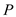is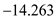indicating that a dollar increase in selling price is expected to decrease sales by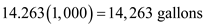in a given sales region. c) We want to test the hypothesis that whether prices are useful in predicting paint sales at 0.05 level of significance. That is, we would perform a statistical test to determine whether the sample value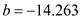is significantly different from zero. The null hypothesis is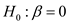, no relationship between sales and price. And, the alternative hypothesis is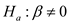, no relationship between sales and price. The standard deviation of error term isSubstitute appropriate values form table.The standard deviation for the sample distribution isAt 5 percent level of significance,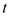-statistic can be calculated asWith 10 observations, a-distribution for the sample statistic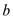will have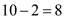degrees of freedom. From Table 2 of Appendix B, the-value is obtained as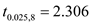The calculated value of t from is less than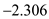, we reject null hypothesis. Thus, we conclude that a linear and negative relationship exists between selling price and paint sales at the 5 percent level of significance. d) The coefficient of determination measures the proportion of total variation in dependent variable explained by the independent variable. That is,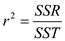. Following table shows the calculation of explained sum of square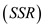, unexplained sum of square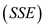and total sum of square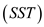.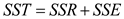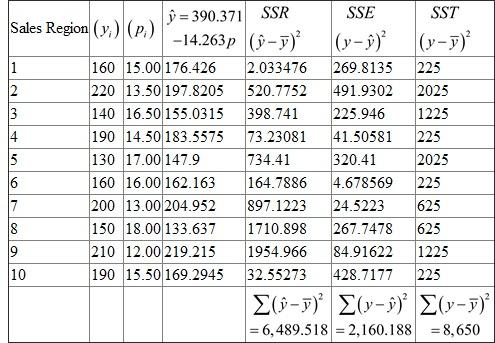Substitute appropriate values from table in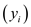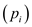Thus, the coefficient of determination is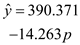. It implies that price as an independent variable explains 75 percent of the variation in paint sales. e) Following are the results of an analysis of variance (ANOVA) on the regression.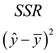The null hypothesis is(no relationship between sales and price) And, the alternative hypothesis is(relationship exists between sales and price) With 10 observations and 1 independent variable, a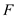-distribution for each level of statistical significance has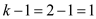degrees of freedom for numerator and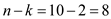degrees of freedom for denominator. (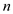is the total number of observations and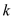is the number of estimated regression parameters, including the constant term) From Table 3 of Appendix B, the-ratio for the above mentioned degrees of freedom at the 5 percent level of significance is 5.32. Thestatistic, however, in the regression model is calculated as 24.034. This value is quite greater than the critical value. Thus, we reject the null hypothesis of no relationship between the paint sales and selling price. f) Substitute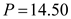in the estimated regression equation to obtain the salesforecast.The best estimate for paint sales in a region, therefore, is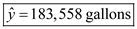. The standard error of the regression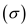as calculated in part (c) is 16.43. Thus, an approximate 95 percent forecast interval of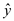isThus, we are 95 percent confident that the true sales of paint at price of \$14.50 per gallon will be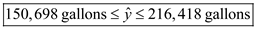. g) The price elasticity of demand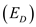measures the responsiveness of change in quantity demanded of paint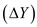to the change in its price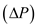. It is calculated as…… (1) whereis the coefficient of sales with respect to price. Substitute,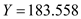and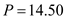in (1)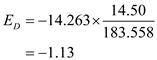The point price elasticity of paint sales is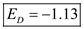.
The data below shows the per capita can consumption of soft drink per year related to price of six packs, per capita income, and mean temperature across the 48 adjoining states in the U.S.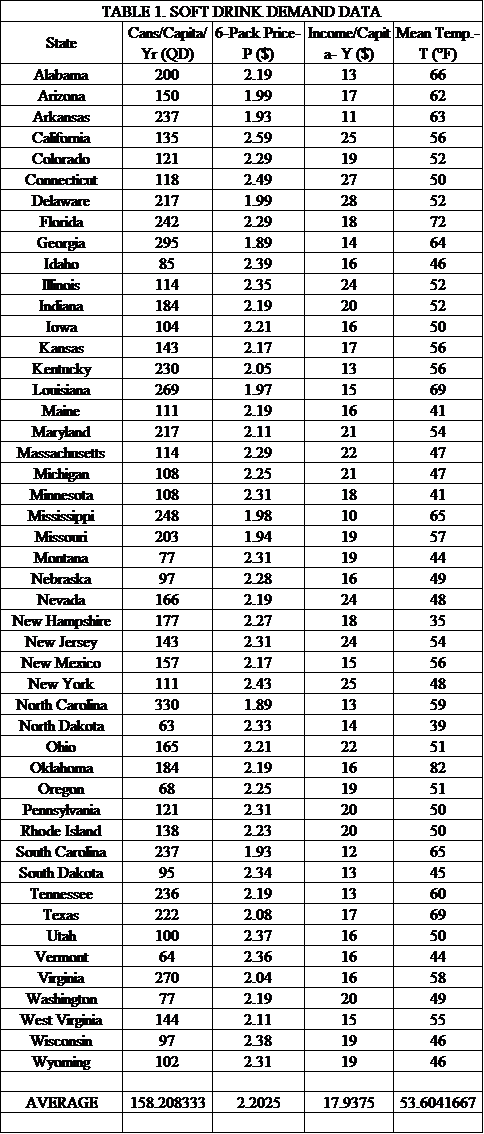• Calculate average cans per capita across 48 contiguous states in the U.S. by entering the formula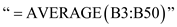in cell B52. • Calculate average 6-pack price across 48 contiguous states in the U.S. by entering the formula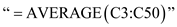in cell C52. The intercept is calculated as 514.267. The coefficients of 6-pack price, income per capita and mean temperature are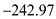, 1.22 and 2.93. Thus, the demand equation estimated using OLS is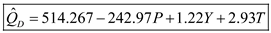. According to the estimated regression equation: • If 6-pack price rises by one unit, the demand for soft drink cans per capita declines by 243 units (holding income and mean temperature constant). • If the per capita income of the increases by one unit, the demand for soft drink cans per capita increases by 1.22 units (holding 6-pack price and mean temperature constant). • Further, if mean temperature increases by one unit, the demand for soft drink cans per capita increases by 2.93 units (holding 6-pack price and income constant). The price elasticity of demandmeasures the responsiveness of change in quantity demanded to change in price. It is calculated as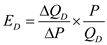…… (1) where,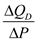is the coefficient of 6-pack price for the estimated demand equation,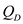is the average demand andis the average price. Substitute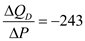,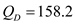and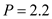in (1)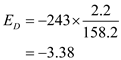Thus, the price elasticity of soft drink demand is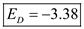.